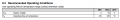# power supply for 4066 analog bilateral switch

#### PeteHL

Joined Dec 17, 2014
340
It is recommended that power supply for the 4066 when used as an analog bilateral switch is +/- 5V. In my circuit that amplitude modulates a sine wave signal, the power supply for that circuit is +/- 8V. Will this degrade operation of the switch, and if so, in what way?

The circuit is my implementation of a cosine burst generator roughly following the design by Linkwitz. The power supply of what he designed is +/- 6V. If interested or if need be I might be willing to describe the circuit in greater detail.

Pete

#### OBW0549

Joined Mar 2, 2015
3,405
It is recommended that power supply for the 4066 when used as an analog bilateral switch is +/- 5V. In my circuit that amplitude modulates a sine wave signal, the power supply for that circuit is +/- 8V. Will this degrade operation of the switch, and if so, in what way?
Looking at the data sheet for a CD4066B, the Recommended Operating Conditions specify a total supply voltage (Vdd - Vss) of 3 to 18 volts:So it would appear that ±8 volts should be OK.

As for degradation, I wouldn't expect any; in fact, with most analog switch ICs, the higher the supply voltage the lower the ON resistance of the switch-- that is, higher supply voltage improves performance, at least with respect to Ron.

#### eetech00

Joined Jun 8, 2013
1,894
It is recommended that power supply for the 4066 when used as an analog bilateral switch is +/- 5V. In my circuit that amplitude modulates a sine wave signal, the power supply for that circuit is +/- 8V. Will this degrade operation of the switch, and if so, in what way?

The circuit is my implementation of a cosine burst generator roughly following the design by Linkwitz. The power supply of what he designed is +/- 6V. If interested or if need be I might be willing to describe the circuit in greater detail.

Pete
Hi

The bipolar supply is needed to pass a bipolar signal. According to the data sheet the max bipolar input signal is 7.5v pk-pk (or 3.75v pk). As long as the input signal does not exceed the recommended..should be ok.

eT

#### Audioguru

Joined Dec 20, 2007
11,249
Hi

The bipolar supply is needed to pass a bipolar signal. According to the data sheet the max bipolar input signal is 7.5v pk-pk (or 3.75v pk).
No. The absolute max input is plus or minus 9V (18V p-p) but plus and minus 7.5V (15V peak-to-peak) is recommended as a max.
A bipolar supply is not needed if the input and output have coupling capacitors and the input is biased at half the supply voltage.

#### PeteHL

Joined Dec 17, 2014
340
hi,
A point to be aware of is the Control Voltage for the 4066 with reference to the supply voltage.
E
View attachment 147639
In the chart, is this assuming that pin 7 of the 4066 is at ground? In other words, the max. control voltage is with respect to supply voltages of 5V, 10V, and 15V?

Then for example where Vdd equals 5V, does the chart mean that the control voltage should not exceed 3.5V with respect to ground, or the voltage at pin 7?

#### PeteHL

Joined Dec 17, 2014
340
@OBW0549 and @dl324

I agree, but then I don't see why Linkwitz and Don Lancaster in his CMOS COOKBOOK specify respectively 12V and 10V supply voltages. In Lancaster's book, the 10V supply is Vdd= +5V and Vss = -5V when routing an analog signal referenced to ground through one of the switches .

Thank you,
Pete

#### Audioguru

Joined Dec 20, 2007
11,249
In the chart, is this assuming that pin 7 of the 4066 is at ground? In other words, the max. control voltage is with respect to supply voltages of 5V, 10V, and 15V?

Then for example where Vdd equals 5V, does the chart mean that the control voltage should not exceed 3.5V with respect to ground, or the voltage at pin 7?
Pin 7 is logic and input ground. Pin 14 is the logic supply and analog maximum positive input voltage. The chart does not show the maximum allowed control voltage. Instead it is the minimum logic high control voltage. An ordinary CD4001 and CD4011 logic IC also has the same minimum logic high input voltage with respect to its supply voltage.

#### OBW0549

Joined Mar 2, 2015
3,405
Then for example where Vdd equals 5V, does the chart mean that the control voltage should not exceed 3.5V with respect to ground, or the voltage at pin 7?
No, that's the minimum voltage at the control pin needed to turn the switch on. The maximum voltage that should be applied to the control pin is the positive supply voltage.

@OBW0549 and @dl324

I agree, but then I don't see why Linkwitz and Don Lancaster in his CMOS COOKBOOK specify respectively 12V and 10V supply voltages. In Lancaster's book, the 10V supply is Vdd= +5V and Vss = -5V when routing an analog signal referenced to ground through one of the switches .
So long as the supply voltages are adequate for the analog signal range, and are within the Absolute Maximum Ratings of the part, the choice between 12V and 10V is entirely up to the designer's preference. The fact that one designer chose 12V and the other 10V, is of no significance.